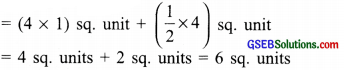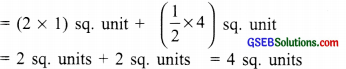# GSEB Solutions Class 6 Maths Chapter 10 Mensuration Ex 10.2

Gujarat Board GSEB Textbook Solutions Class 6 Maths Chapter 10 Mensuration Ex 10.2 Textbook Questions and Answers.

## Gujarat Board Textbook Solutions Class 6 Maths Chapter 10 Mensuration Ex 10.2

Question 1.
Find the area of the following figures by counting squaresSolution:
(a) Number of full squares = 9
∵ Area of 1 square = 1 sq. unit
∴ Area of the portion covered by the figure = 9 × 1 sq. unit = 9 sq. units

(b) ∵ Number of full squares = 5
∴ Area of the figure = 5 × 1 sq. unit = 5 sq. units

(c) Number of full squares = 2
Half squares = 4
∴ Area covered by the figure(d) Number of full squares = 8
∴ Area covered by the figure
= 8 × 1 sq. unit = 8 sq. units

(e) ∵ Number of full squares = 10
∴ Area covered by the figure
= 10 × 1 sq. unit = 10 sq. units

(f) ∵ Number of full squares = 2
Half squares = 4
∴ Area of the figure= 2 sq. units + 2 sq. units
= 4 sq. units

(g) Number of full squares = 4
Half squares = 4
∴ Area of the figure(h) ∵ Number of full squares = 5
∴ Area of the figure
= 5 × 1 sq. unit = 5 sq. units

(i) ∵ Number of full squares = 9
∴ Area of the figure = 9 × 1 sq. unit = 9 sq. units(j) ∵ Number of full squares = 2
Half squares = 4
∴ Area of figure(k) ∵ Number of full squares = 4
Half squares = 2
∴ Area of the figure
= 4 × 1 sq. unit + $$\frac { 1 }{ 2 }$$ × 2 sq. unit
= 4 sq. units + 1 sq. unit = 5 sq. units

(l) ∵ Number of full squares = 4
More than half squares = 3
Half squares = 2
∴ Area of the figure= 4 sq. units + 3 sq. units + 2 sq. unit = 9 sq. units

(m) ∵ Number of full squares = 6
More than half squares = 8
Half squares = 0
∴ Area covered by the figure = (6 × 1) sq. unit + (8 × 1) sq. unit
= 6 sq. units + 8 sq. units = 14 sq. units

(n) ∵ Number of full squares = 9
More than half squares = 9
∴ Area of the figure
= (9 × 1) sq. unit + (9 × 1) sq. unit = 9 sq. units + 9 sq. units = 18 sq. units